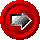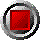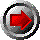GenCurveBondPriceRVOddFirstHol() functionGenCurveBondPriceRVOddFirstHol() function
GenCurveBondPriceRVOddFirstHol(argument list…)

This function prices a bond with an odd first coupon (short or long) using a zero curve. The zero curve can be defined either by zero rates or discount factors, and different interpolation methods are offered. Cashflows are adjusted to the next valid business day using the holiday array, if they happen to fall on weekends or holidays. The function uses the following arguments:

 Argument Description Restrictions Valuation_Date valuation date (e.g. today) valid Excel date number Settlement_Date bond settlement date valid Excel date number >= Valuation_Date >= Issue_Date Maturity_Date bond maturity date valid Excel date number >= Settlement_Date > Issue_Date > First_Coup_Date Issue_Date the issue date of the bond < First_Coup_Date First_Coup_Date the first coupon date of the bond same coupon cycle as Maturity_Date (i.e. consistent with Freq argument) Coupon annual bond coupon in decimal form (e.g. six percent entered as 0.06). For zero coupon (strip) bonds, enter 0. >= 0 Freq number of bond coupons per annum 1, 2, 4, or 12 DCB day count basis 0 = 30/360 (US) 1 = act/act for US T-Bonds 2 = act/360 3 = act/365 4 = 30/360 (European) 5 = Canadian modified act/365 Redemption redemption value of bond per \$100 par typically \$100 Holidays array of holiday dates valid Excel date numbers strictly ascending order Curve_Type defines how the Zero_Rates array is to be interpreted 0 = continuously compounded riskless rates in, decimal form 1 = discount factors (first must be 1.0, and must be declining) Interpolation the interpolation method to employ for the Zero_Rates array 0 = cubic-spline 1 = linear 2 = log-linear Zero_Dates array of zero coupon curve dates strictly ascending order The first date of this array must be Valuation_Date Zero_Rates an array of zero rates or discount factors corresponding to Zero_Dates OAS parallel shift of the zero curve in decimal form Bucket_Start beginning of bucket for zero curve shifts set to 0 if curve shift is not desired Bucket_End end of bucket for zero curve shift >= Bucket_Start Bucket_Shift parallel shift of the zero curve between Bucket_Start and Bucket_End in decimal form set to 0 if curve shock is not desired

© 1995-98 Leap of Faith Research Inc.